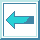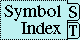#B

backquote n. the standard character that is variously called ``grave accent'' or ``backquote'' (`). See Figure 2-5.

backslash n. the standard character that is variously called ``reverse solidus'' or ``backslash'' (\). See Figure 2-5.

before method n. a method having the qualifier :before.

bidirectional adj. (of a stream) being both an input stream and an output stream.

binary adj. 1. (of a stream) being a stream that has an element type that is a subtype of type integer. The most fundamental operation on a binary input stream is read-byte and on a binary output stream is write-byte. See character. 2. (of a file) having been created by opening a binary stream. (It is implementation-dependent whether this is an detectable aspect of the file, or whether any given character file can be treated as a binary file.)

bind v.t. (a variable) to establish a binding for the variable.

binding n. an association between a name and that which the name denotes. ``A lexical binding is a lexical association between a name and its value.'' When the term binding is qualified by the name of a namespace, such as ``variable'' or ``function,'' it restricts the binding to the indicated namespace, as in: ``let establishes variable bindings.'' or ``let establishes bindings of variables.''

bit n. an object of type bit; that is, the integer 0 or the integer 1.

bit array n. a specialized array that is of type (array bit), and whose elements are of type bit.

bit vector n. a specialized vector that is of type bit-vector, and whose elements are of type bit.

bit-wise logical operation specifier n. an object which names one of the sixteen possible bit-wise logical operations that can be performed by the boole function, and which is the value of exactly one of the constant variables boole-clr, boole-set, boole-1, boole-2, boole-c1, boole-c2, boole-and, boole-ior, boole-xor, boole-eqv, boole-nand, boole-nor, boole-andc1, boole-andc2, boole-orc1, or boole-orc2.

block n. a named lexical exit point, established explicitly by block or implicitly by operators such as loop, do and prog, to which control and values may be transfered by using a return-from form with the name of the block.

block tag n. the symbol that, within the lexical scope of a block form, names the block established by that block form. See return or return-from.

boa lambda list n. a lambda list that is syntactically like an ordinary lambda list, but that is processed in ``by order of argument'' style. See Section 3.4.6 (Boa Lambda Lists).

body parameter n. a parameter available in certain lambda lists which from the point of view of conforming programs is like a rest parameter in every way except that it is introduced by &body instead of &rest. (Implementations are permitted to provide extensions which distinguish body parameters and rest parameters---e.g., the forms for operators which were defined using a body parameter might be pretty printed slightly differently than forms for operators which were defined using rest parameters.)

boolean n. an object of type boolean; that is, one of the following objects: the symbol t (representing true), or the symbol nil (representing false). See generalized boolean.

boolean equivalent n. (of an object O1) any object O2 that has the same truth value as O1 when both O1 and O2 are viewed as generalized booleans.

bound adj., v.t. 1. adj. having an associated denotation in a binding. ``The variables named by a let are bound within its body.'' See unbound. 2. adj. having a local binding which shadows another. ``The variable *print-escape* is bound while in the princ function.'' 3. v.t. the past tense of bind.

bound declaration n. a declaration that refers to or is associated with a variable or function and that appears within the special form that establishes the variable or function, but before the body of that special form (specifically, at the head of that form's body). (If a bound declaration refers to a function binding or a lexical variable binding, the scope of the declaration is exactly the scope of that binding. If the declaration refers to a dynamic variable binding, the scope of the declaration is what the scope of the binding would have been if it were lexical rather than dynamic.)

bounded adj. (of a sequence S, by an ordered pair of bounding indices istart and iend) restricted to a subrange of the elements of S that includes each element beginning with (and including) the one indexed by istart and continuing up to (but not including) the one indexed by iend.

bounding index n. (of a sequence with length n) either of a conceptual pair of integers, istart and iend, respectively called the ``lower bounding index'' and ``upper bounding index'', such that 0 <=istart <=iend <=n, and which therefore delimit a subrange of the sequence bounded by istart and iend.

bounding index designator (for a sequence) one of two objects that, taken together as an ordered pair, behave as a designator for bounding indices of the sequence; that is, they denote bounding indices of the sequence, and are either: an integer (denoting itself) and nil (denoting the length of the sequence), or two integers (each denoting themselves).

break loop n. A variant of the normal Lisp read-eval-print loop that is recursively entered, usually because the ongoing evaluation of some other form has been suspended for the purpose of debugging. Often, a break loop provides the ability to exit in such a way as to continue the suspended computation. See the function break.

built-in class n. a class that is a generalized instance of class built-in-class.

built-in type n. one of the types in Figure 4-2.

byte n. 1. adjacent bits within an integer. (The specific number of bits can vary from point to point in the program; see the function byte.) 2. an integer in a specified range. (The specific range can vary from point to point in the program; see the functions open and write-byte.)

byte specifier n. An object of implementation-dependent nature that is returned by the function byte and that specifies the range of bits in an integer to be used as a byte by functions such as ldb.

The following X3J13 cleanup issue, not part of the specification, applies to this section: